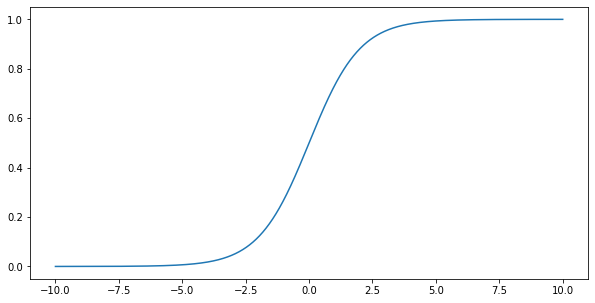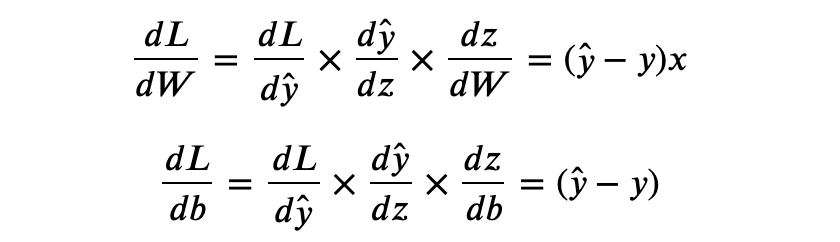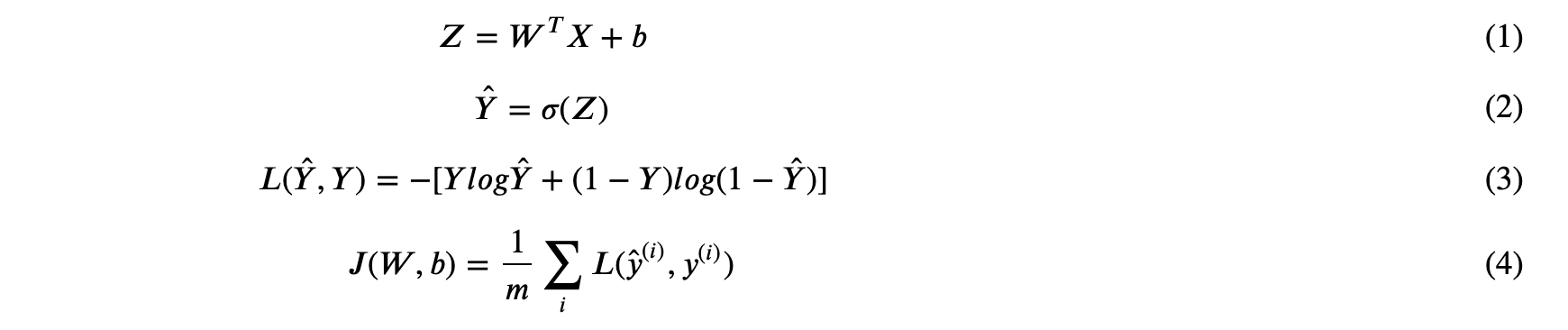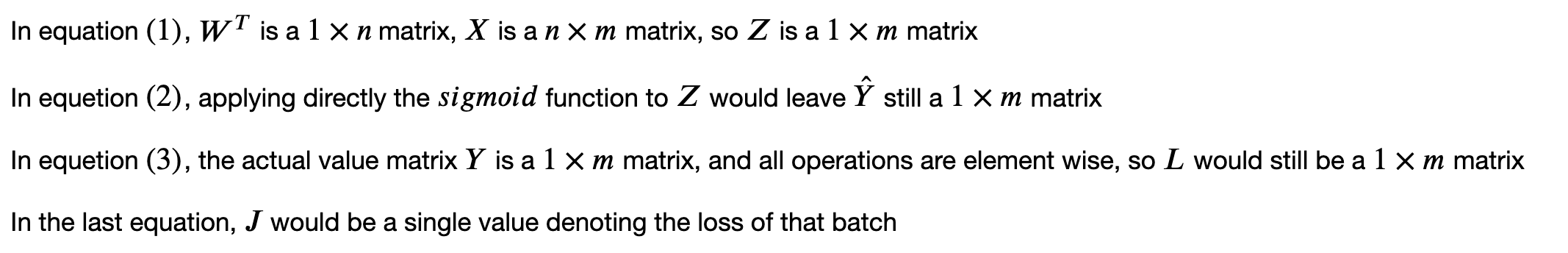# Logistic Regression Step by Step ImplementationLogistic regression is a statistical model that in its basic form uses a logistic function to model a binary dependent variable, although many more complex extensions exist. In regression analysis, logistic regression (or logit regression) is estimating the parameters of a logistic model (a form of binary regression)

Say we are doing a classic prediction task, where given a input vector with \$n\$ variables:And to predict 1 response variable \$y\$ (may be the sales of next year, the house price, etc.), the simplest form is to use a linear regression to do the prediction with the formula:Where \$W\$ is a column vector with \$n\$ dimension. Say now our question changed a bit, we hope to predict a probability, like what’s the probability of raining tomorrow? In this sense, this linear regression might be a little unfit here, as a linear expression can be unbounded but our probability is ranged in \$[0, 1]\$.

## Sigmoid Function

To bound our prediction in \$[0, 1]\$, the widely used technic is to apply a `sigmoid` function:With `numpy` we can easily visualize the function.## Loss Function

The definition of loss function of logistic regression is:Where `y_hat` is our prediction ranging from \$[0, 1]\$ and `y` is the true value. When the actual value is `y = 1`, the equation becomes:the closer `y_hat` to 1, the smaller our loss is. And the same goes for `y = 0` .

Given this actual value `y`, we hope to minimize the loss `L`, and the technic we are going to apply here is gradient descent(the details has been illustrated here), basically what we need to do is to apply derivative to our variables and move them slightly down to the optimum.

Here we have 2 variables, `W` and `b`, and for this example, the update formula of them would be:Where `W` is a column vector with `n` weights correspond to the `n` dimension of `x^(i)`. In order to get the derivative of our targets, chain rules would be applied:You can try out the deduction on your own, the only tricky part is the derivative of `sigmoid` function, for a good explanation you can refer to here.

## Batch Training

The above gives the forward and backward updating process, which is well enough to implement a logistic regression if we were to feed in our training model ONE AT A TIME. However, in most training cases, we don’t do that. Instead training samples are feed in batches, and the backward propagation is updated with average loss of the batch.

Which means that for a model that feed with `m` samples at a time, the loss function would be:Where `i` denotes the `ith` training sample.

## Forward Propagation of Batch Training

Now instead of using `x`, a single vector, as our input, we specify a matrix `X` with size `n x m`, where as above, `n` is the number of features and `m` is number of training samples (basically, we line up `m` training samples in a matrix). Now the formula becomes:Note that here we use UPPER LETTER to denote our matrix and vectors (a caveat is that `b` here is still a single value, the more formal way would be to represent `b` as a vector, but in python the addition of a single value to a matrix would be automated broadcasted).

Let’s break down the size of the matrices one by one.Our formula stuff ends here, let’s implement our algorithm, before that some data needs to be generated to make a classification task (the whole implementation is also in my git repo).

``````from sklearn import datasets

X, y = datasets.make_classification(n_samples=1000, random_state=123)

X_train, X_test = X[:700], X[700:]
y_train, y_test = y[:700], y[700:]``````

## Hire Machine Learning Developers in India

We supply you with world class machine learning experts / ML Developers with years of domain experience who can add more value to your business.

## What is Supervised Machine Learning

What is neuron analysis of a machine? Learn machine learning by designing Robotics algorithm. Click here for best machine learning course models with AI

## Pros and Cons of Machine Learning Language

AI, Machine learning, as its title defines, is involved as a process to make the machine operate a task automatically to know more join CETPA

## Top 10 Deep Learning Sessions To Look Forward To At DVDC 2020

Looking to attend an AI event or two this year? Below ... Here are the top 22 machine learning conferences in 2020: ... Start Date: June 10th, 2020 ... Join more than 400 other data-heads in 2020 and propel your career forward. ... They feature 30+ data science sessions crafted to bring specialists in different ...

## Machine Learning in Java With Amazon Deep Java Library

Machine Learning in Java With Amazon Deep Java Library .In this article, we demonstrate how Java developers can use the JSR-381 VisRec API to implement image classification or object detection with DJL’s pre-trained models in less than 10 lines of code.